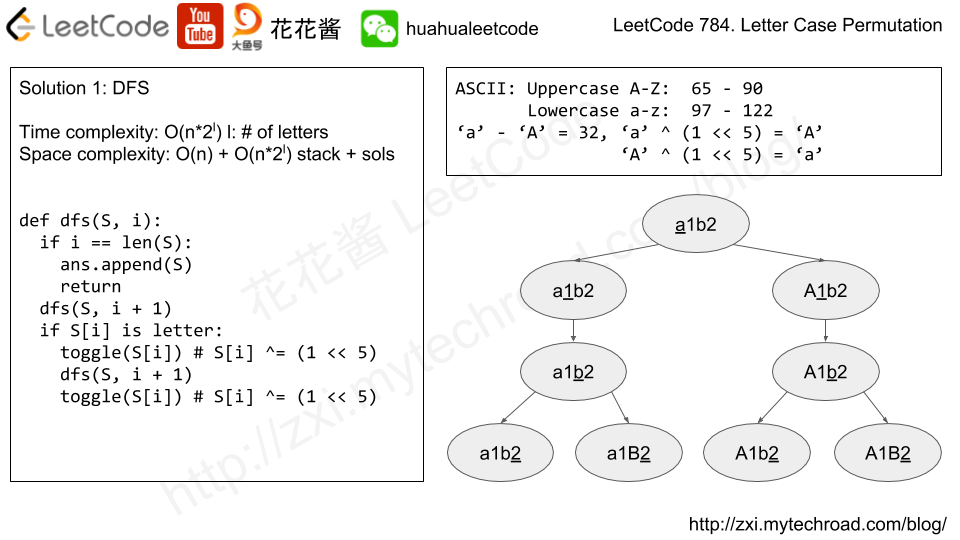# Problem

Implement next permutation, which rearranges numbers into the lexicographically next greater permutation of numbers.

If such arrangement is not possible, it must rearrange it as the lowest possible order (ie, sorted in ascending order).

The replacement must be in-place and use only constant extra memory.

Here are some examples. Inputs are in the left-hand column and its corresponding outputs are in the right-hand column.

1,2,3 → 1,3,2
3,2,1 → 1,2,3
1,1,5 → 1,5,1

# Solution

Find the last acceding element x, swap with the smallest number y, y is after x that and y is greater than x.

Reverse the elements after x.

Time complexity: O(n)

Space complexity: O(1)

# Problem

Given a collection of numbers that might contain duplicates, return all possible unique permutations.

Example:

Input: [1,1,2]
Output:
[
[1,1,2],
[1,2,1],
[2,1,1]
]

# Solution

Time complexity: O(n!)

Space complexity: O(n + k)

# Problem

Given a non-negative integer n, count all numbers with unique digits, x, where 0 ≤ x < 10n.

Example:
Given n = 2, return 91. (The answer should be the total numbers in the range of 0 ≤ x < 100, excluding [11,22,33,44,55,66,77,88,99])

# Solution: Math

f(0) = 1 (0)

f(1) = 10 (0 – 9)

f(2) = 9 * 9 (1-9 * (0 ~ 9 exclude the one from first digit))

f(3) = 9 * 9 * 8

f(4) = 9 * 9 * 8 * 7

f(x) = 0 if x >= 10

ans = sum(f ~ f[n])

Time complexity: O(1)

Space complexity: O(1)

Shuffle a set of numbers without duplicates.

Example:

C++

Given a string S, we can transform every letter individually to be lowercase or uppercase to create another string.  Return a list of all possible strings we could create.

Note:

• S will be a string with length at most 12.
• S will consist only of letters or digits.# Solution 1: DFS

Time complexity: O(n*2^l), l = # of letters in the string

Space complexity: O(n) + O(n*2^l)

## Python 3

Mission News Theme by Compete Themes.